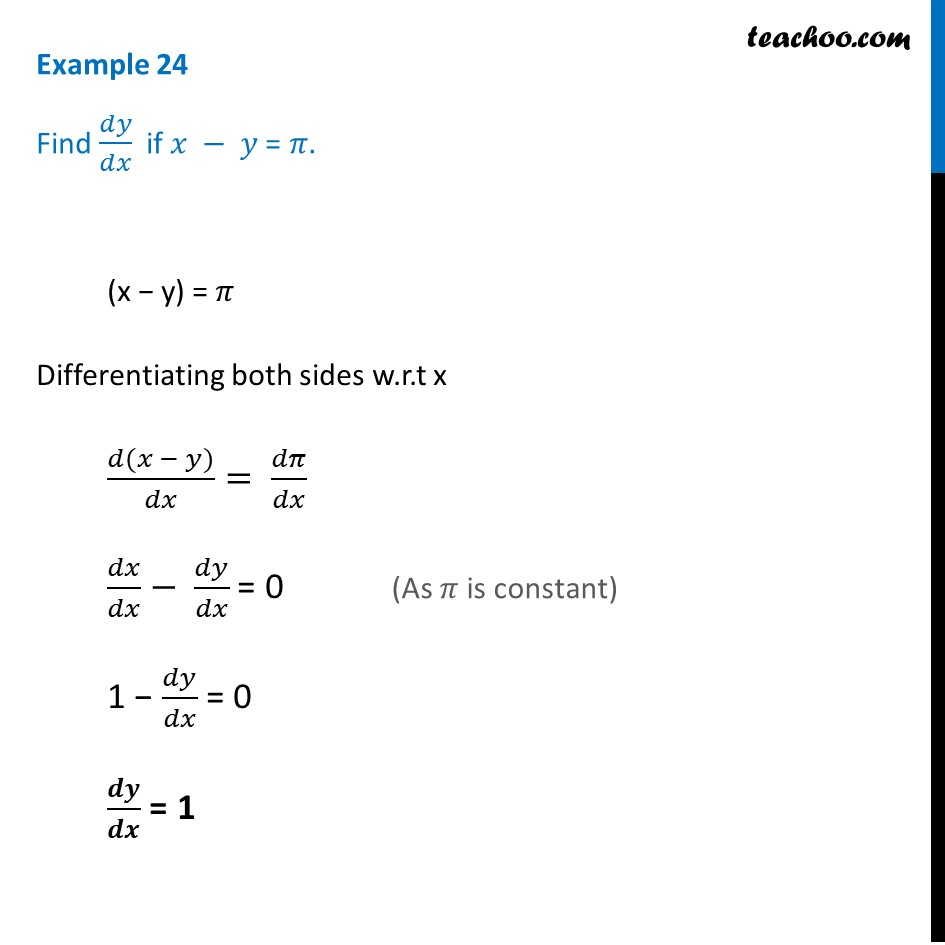1. Chapter 5 Class 12 Continuity and Differentiability (Term 1)
2. Concept wise
3. Finding derivative of Implicit functions

Transcript

Example 24 Find 𝑑𝑦/𝑑𝑥 if 𝑥 − 𝑦 = 𝜋. (x − y) = 𝜋 Differentiating both sides w.r.t x (𝑑(𝑥 − 𝑦))/𝑑𝑥= 𝑑𝜋/𝑑𝑥 𝑑𝑥/𝑑𝑥− 𝑑𝑦/𝑑𝑥 = 0 1 − 𝑑𝑦/𝑑𝑥 = 0 𝒅𝒚/𝒅𝒙 = 1 (As 𝜋 is constant)